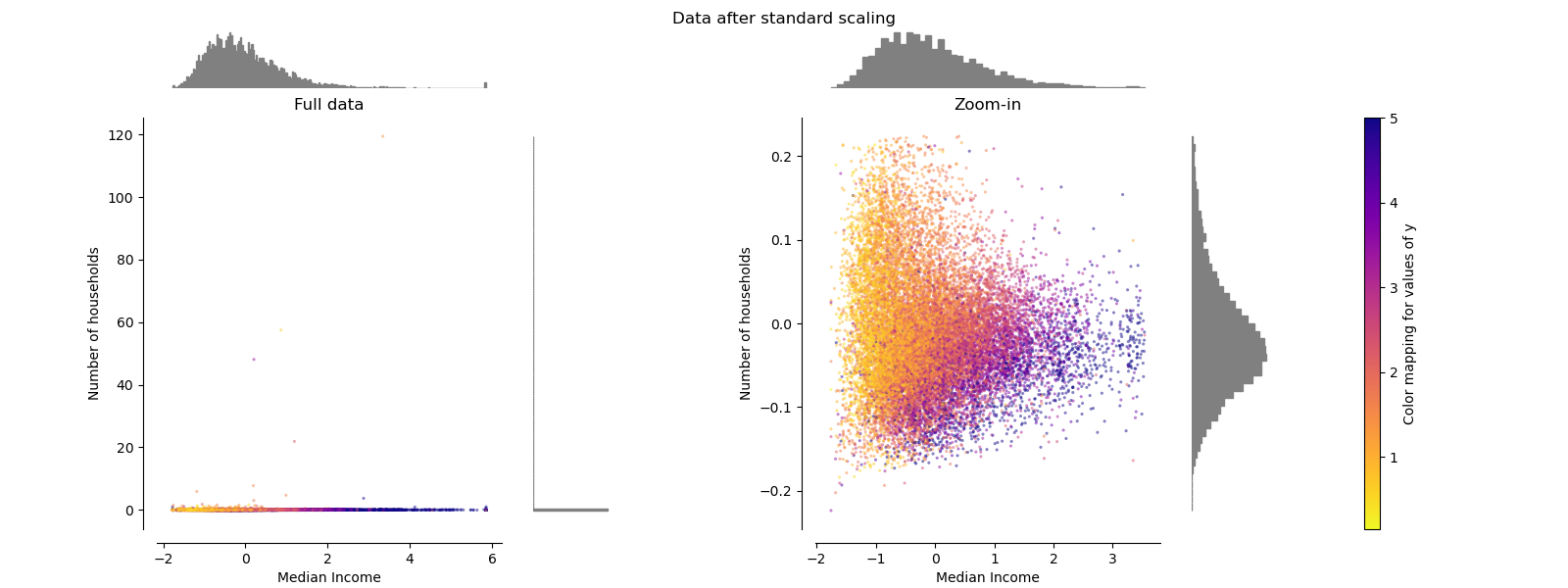# sklearn.preprocessing.MaxAbsScaler¶

class sklearn.preprocessing.MaxAbsScaler(*, copy=True)

[源码]

copy boolean, optional, default is True

scale_ ndarray, shape (n_features,)

0.17版中的新功能：scale_属性。
max_abs_ ndarray, shape (n_features,)

n_samples_seen_ int

maxabs_scale

NaN被视为缺失值：忽略适合度，并保持变换值。

>>> from sklearn.preprocessing import MaxAbsScaler>>> X = [[ 1., -1.,  2.],...      [ 2.,  0.,  0.],...      [ 0.,  1., -1.]]>>> transformer = MaxAbsScaler().fit(X)>>> transformerMaxAbsScaler()>>> transformer.transform(X)array([[ 0.5, -1. ,  1. ],       [ 1. ,  0. ,  0. ],       [ 0. ,  1. , -0.5]])

fit(X[, y]) 计算用于以后缩放的最大绝对值。
fit_transform(X[, y]) 拟合数据，然后对其进行转换。
get_params([deep]) 获取此估计量的参数。
inverse_transform(X) 将数据按比例缩小到原始表示形式
partial_fit(X[, y]) 在线计算X的最大绝对值，以便以后缩放。
set_params(**params) 设置此估算器的参数。
transform(X) 缩放数据
__init__(*, copy=True)

[源码]

fit(X, y=None)

[源码]

X {array-like, sparse matrix}, shape [n_samples, n_features]

fit_transform(X, y=None, **fit_params)

[源码]

X {array-like, sparse matrix, dataframe} of shape (n_samples, n_features)
y ndarray of shape (n_samples,), default=None

**fit_params dict

X_new ndarray array of shape (n_samples, n_features_new)

get_params(deep=True)

[源码]

deep bool, default=True

params mapping of string to any

inverse_transform(X)

[源码]

X {array-like, sparse matrix}

partial_fit(X, y=None)

[源码]

X {array-like, sparse matrix}, shape [n_samples, n_features]

y None

set_params(**params)

[源码]

**params dict

self object

transform(X)

[源码]

X {array-like, sparse matrix}

## sklearn.preprocessing.MaxAbsScaler使用示例¶